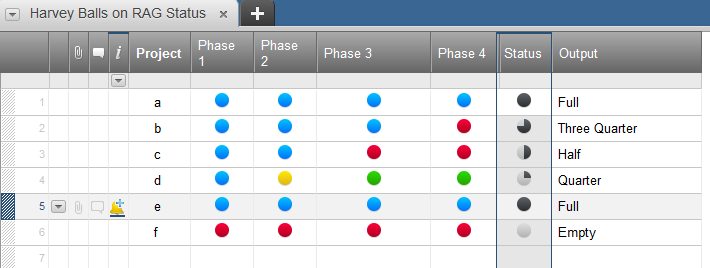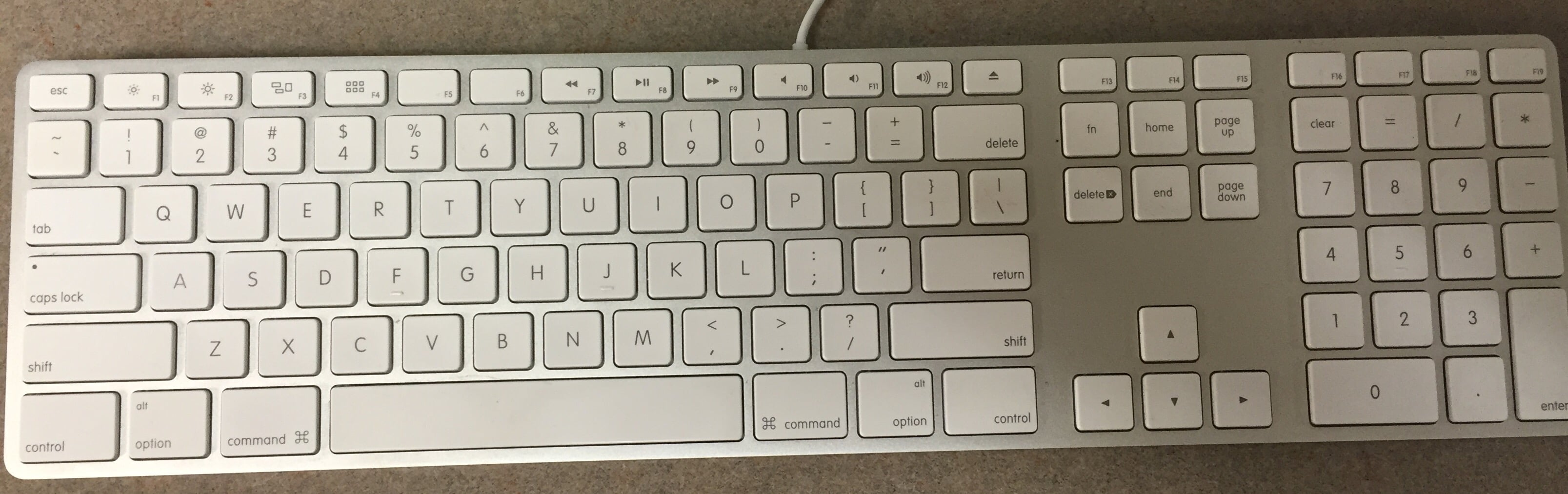#### Welcome to the Smartsheet Forum Archives

The posts in this forum are no longer monitored for accuracy and their content may no longer be current. If there's a discussion here that interests you and you'd like to find (or create) a more current version, please Visit the Current Forums.

# Automating Harvey Balls based on RAG status

Hi,

Could somoen please help with the formula I need to use to automate completion of Harvey Balls based on RAG status of four phases?

I am using RAG status balls (Green, Yellow, Red and Blue) to indicate status of each phase of the implementation.  I have four implementation phases and basically want the Harvey Balls to Go from Quarter full when I have Blue RAG for phase 1, to Half full when I have Blue RAG for phase 2 etc. all the way to full when I have Blue RAG agains all four phases of my implementation (we chose Blue to denominate completion of the phase).

I tried to use the nested IF formual that you have on your Formula Example sheet but I keep getting an error :-(

Any help would be much appreciated.

• I hope I am understanding your need.  Try using a formula similar to this.  I put it in the Status column in the image below.

=IF(COUNTIF([Phase 1]1:[Phase 4]1, "Blue") = 0, "Empty", IF(COUNTIF([Phase 1]1:[Phase 4]1, "Blue") = 1, "Quarter", IF(COUNTIF([Phase 1]1:[Phase 4]1, "Blue") = 2, "Half", IF(COUNTIF([Phase 1]1:[Phase 4]1, "Blue") = 3, "Three Quarter", "Full"))))

Basically I used a countif function that would count how many blue balls there were, and the then kicked out the status based on that count.

Good luck, and hope this helps.• Joel,

Thanks for your message.  This is exactly what I need.  However I still get #UNPARSEABLE error.  Do you paste the formula in Status column or Output column?  I am assuming that the Status Column should be formated as Symbols?

For whatever reason it is not quite working for me :-(

• I get to the point that I get the Output formula working however it does not change the column with Harvey Balls.

I can see that you have hidden column - does it contain formula/conditional formating that poupulates with an appropriate Harvey Balls depending on the text in Outpu column?

• Hello,

The Formula is in the status column.

What I would typically do is put the formula into the column with it still a 'text' column type.  This will let you see easily if the calculation is working or not.  The text that should appear should be what you see in the 'Output Column'.  Once the text is working properly, then change the column type to symbols with the Harvey Balls.

I did have hidden fields but they were not affecting the equation.

It may be easier touble shooting if you do use two columns.  Make a text column named 'Calc'.

The 'Calc' column simply counts the blue balls using this equation:

=COUNTIF([Phase 1]1:[Phase 4]1, "Blue")

Then in your status Column use the formula:

=IF(Calc1 = 0, "Empty", IF(Calc1 = 1, "Quarter", IF(Calc1 = 2, "Half", IF(Calc1 = 3, "Three Quarter", "Full"))))

This esentially does the same thing but you can see it counting.  Let me know if this helps.

• I have published the sheet so you can see it work, and also copy from it, and edit it if you need.  Here is the link:

https://app.smartsheet.com/b/publish?EQBCT=a91c2d50ae2f4c6786ebdc41349d596d

I'll keep it up for a few days.  Thanks.

• OMG! This si so extremely helpful. Thank you so very much.

• Hi Joel,

I hope you don't mind, but I also accessed your sheet to see the formula and how it works within your sheet.

I've used Smartsheet for some time, but haven't really taken the opportunity to dive into formulas and other capabilities.  This is me dipping my toes in the water with formulas, which I thought was pretty basic.  For some reason I keep getting the same #UNPARSEABLE error message when I try to nest all 4 IF formulas together. Would you be willing to look it over and see that it's correct?  I even color coded it so I could see each section was correct, but I'm not seeing the error in the below formula...

=IF([Sales Stage]27 = "1 - Prospecting", “Yellow” , IF([Sales Stage]27 = "2 - Proposal", “Blue”, IF([Sales Stage]27 = "3 - Contracts", “Green”, IF([Sales Stage]27 = "4 - Lost", “Red”))))

I appreciate any information and guidance!

• Valerie - you are getting the error because half the quotes are in the incorrect format.

Quotes should be this: "

Not: “ ”

Try this:

=IF([Sales Stage]27 = "1 - Prospecting", "Yellow", IF([Sales Stage]27 = "2 - Proposal", "Blue", IF([Sales Stage]27 = "3 - Contracts", "Green", IF([Sales Stage]27 = "4 - Lost", "Red"))))

• Hi Travis,

Thank you so much for your assistance. The corrected formula worked great!

I have a MAC and use this keyboard. I don't see differing " other than the one by the return key. Is there another way I should use the " in doing formulas on Smartsheet?• I use a Mac as well! There is not a different key for quotes. Did you copy and paste any part of the formula from a word processor, Help Center article, or another source? Some applications or sources will use rich text quotes (rather than the straight line quotes) and if it is copied into Smartsheet, it will not work.

For example, if you build the formula in Microsoft Word, you will get rich text quotes and the formula will not work in Smartsheet.

Best bet is to build the formula directly in Smartsheet or just keep an eye on the quotes if you are copying and pasting from another source.

• Travis, That's exactly what happened !  Thank you so much for taking the time to clarify and help me figure out what was happening. Have a great day!

• Happy to help!This discussion has been closed.